JMSLTM Numerical Library 7.2.0
com.imsl.datamining

## Class CrossValidation

• All Implemented Interfaces:
Serializable, Cloneable

```public class CrossValidation
extends Object
implements Serializable, Cloneable```
Performs V-Fold cross-validation for predictive models. In V-fold cross validation, the training data is partitioned randomly into V approximately equally sized sub-samples. The model is then trained V different times with each of the sub-samples removed in turn. The cross-validated estimate of the risk function is given by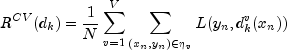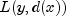is the loss incurred when the prediction is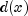for the actual y. The inner summation is over the examples in the test sample held out for each fold.

If the predictive model is an instance of a decision tree, cross-validation is performed on each optimal sub-tree determined by cost-complexity pruning. Let the symbol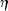denote the full training data set,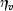the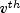sub-sample. Then use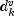to indicate the set of predictions corresponding to the kth optimal sub-tree fitted on the training sample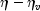. To select one sub-tree from among the configurations, two criteria are the minimum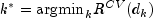and the least complicated (smallest sub-tree) which satisfies: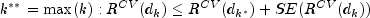The standard error is approximated by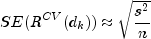with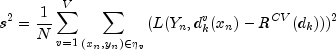The summation is over each fold and each learning sub-sample within each fold.

Example 1, Serialized Form
• ### Constructor Summary

Constructors
Constructor and Description
`CrossValidation(PredictiveModel pm)`
Creates a `CrossValidation` object.
• ### Method Summary

Methods
Modifier and Type Method and Description
`void` `crossValidate()`
Performs V-Fold cross-validation.
`double` `getCrossValidatedError()`
Returns the cross-validated error.
`int` `getNumberOfSampleFolds()`
Returns the number of folds set for the cross validation selection.
`int` `getNumberOfThreads()`
Returns the maximum number of `java.lang.Thread` instances that may be used for parallel processing.
`double[]` `getRiskStandardErrors()`
Returns the estimated standard errors for the risk values.
`double[]` `getRiskValues()`
Returns the vector of risk values.
`void` `setNumberOfSampleFolds(int nFolds)`
Sets the number of folds to use in cross validation selection.
`void` `setNumberOfThreads(int numberOfThreads)`
Sets the maximum number of `java.lang.Thread` instances that may be used for parallel processing.
`void` `setRandomObject(Random r)`
Sets the random object to be used in the permutation of observation data.
• ### Methods inherited from class java.lang.Object

`clone, equals, finalize, getClass, hashCode, notify, notifyAll, toString, wait, wait, wait`
• ### Constructor Detail

• #### CrossValidation

```public CrossValidation(PredictiveModel pm)
throws PredictiveModel.PredictiveModelException```
Creates a `CrossValidation` object.
Parameters:
`pm` - an object of a class that extends `PredictiveModel`.
Throws:
`PredictiveModel.PredictiveModelException`
• ### Method Detail

• #### crossValidate

```public void crossValidate()
throws PredictiveModel.PredictiveModelException,
NoSuchMethodException,
InstantiationException,
IllegalAccessException,
InvocationTargetException```
Performs V-Fold cross-validation.
Throws:
`PredictiveModel.PredictiveModelException` - an exception has occurred in the common `PredictiveModel` programming interface methods or an exception class that has extended the `PredictiveModelException` class.
`NoSuchMethodException` - the PredictiveModel subclass is missing a constructor with the expected signature (see com.imsl.datamining.PredictiveModel.PredictiveModel).
`InstantiationException` - the object has failed to instantiate. This maybe have occurred if your PredictiveModel subclass is not concrete.
`IllegalAccessException` - the currently executing method does not have access to the definition of the specified class, field, method or constructor.
`InvocationTargetException` - an exception has occurred within one of the method. Use java.lang.Throwable.getCause() to extract the initiating exception.
• #### getCrossValidatedError

```public double getCrossValidatedError()
throws PredictiveModel.StateChangeException```
Returns the cross-validated error. If the response variable is categorical, the error is the misclassification rate, weighted by the misclassification costs and prior probabilities, attributes of the `PredictiveModel` object. If the response variable is quantitative/continuous, the error is the mean squared prediction error, also weighted if weights are set in the `PredictiveModel` object.
Returns:
a `double` equal to the cross-validated prediction error.
Throws:
`PredictiveModel.StateChangeException` - an input parameter in the `PredictiveModel` changed that might affect the model estimates or predictions.
• #### getNumberOfSampleFolds

`public int getNumberOfSampleFolds()`
Returns the number of folds set for the cross validation selection.
Returns:
an `int` that indicates the number of folds.

`public int getNumberOfThreads()`
Returns the maximum number of `java.lang.Thread` instances that may be used for parallel processing.
Returns:
an `int` containing the maximum number of `java.lang.Thread` instances that may be used for parallel processing.

The actual number of threads used in parallel processing will be the lesser of `numberOfThreads` and `nFolds`, the number of folds set for cross-validation. This assessment is made to optimize use of resources.

• #### getRiskStandardErrors

`public double[] getRiskStandardErrors()`
Returns the estimated standard errors for the risk values.

In most cases the length is 1. For `DecisionTree`, `CrossValidation` returns an array of length >= 1.

Returns:
a `double` array containing the estimated standard errors for the risk values.
• #### getRiskValues

`public double[] getRiskValues()`
Returns the vector of risk values.

In most cases the length is 1. For `DecisionTree`, `CrossValidation` returns an array of length >= 1.

Returns:
a `double` array containing the estimated risk values.
• #### setNumberOfSampleFolds

`public void setNumberOfSampleFolds(int nFolds)`
Sets the number of folds to use in cross validation selection.
Parameters:
`nFolds` - an `int` that specifies the number of folds to use in cross validation selection.

`nFolds` must be between 1 and the number of observations (`xy.length`), inclusive. If `nFolds` = 1 the full data set is used once to generate the `PredictiveModel`. In other words, no cross-validation is performed. If 1 < `xy.length`/`nFolds`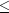3, leave-one-out cross validation is performed.

Default: `nFolds` = 10.

`public void setNumberOfThreads(int numberOfThreads)`
Sets the maximum number of `java.lang.Thread` instances that may be used for parallel processing.
Parameters:
`numberOfThreads` - an `int` specifying the maximum number of `java.lang.Thread` instances that may be used for parallel processing.

The actual number of threads used in parallel processing will be the lesser of `numberOfThreads` and `nFolds`, the number of folds set for cross-validation. This assessment is made to optimize use of resources.

Default: `numberOfThreads` = 1.

• #### setRandomObject

`public void setRandomObject(Random r)`
Sets the random object to be used in the permutation of observation data.
Parameters:
`r` - a `Random` object to be used in random permutation of observation data.

Specifying a seed for the `Random` object can produce repeatable/deterministic output.

JMSLTM Numerical Library 7.2.0